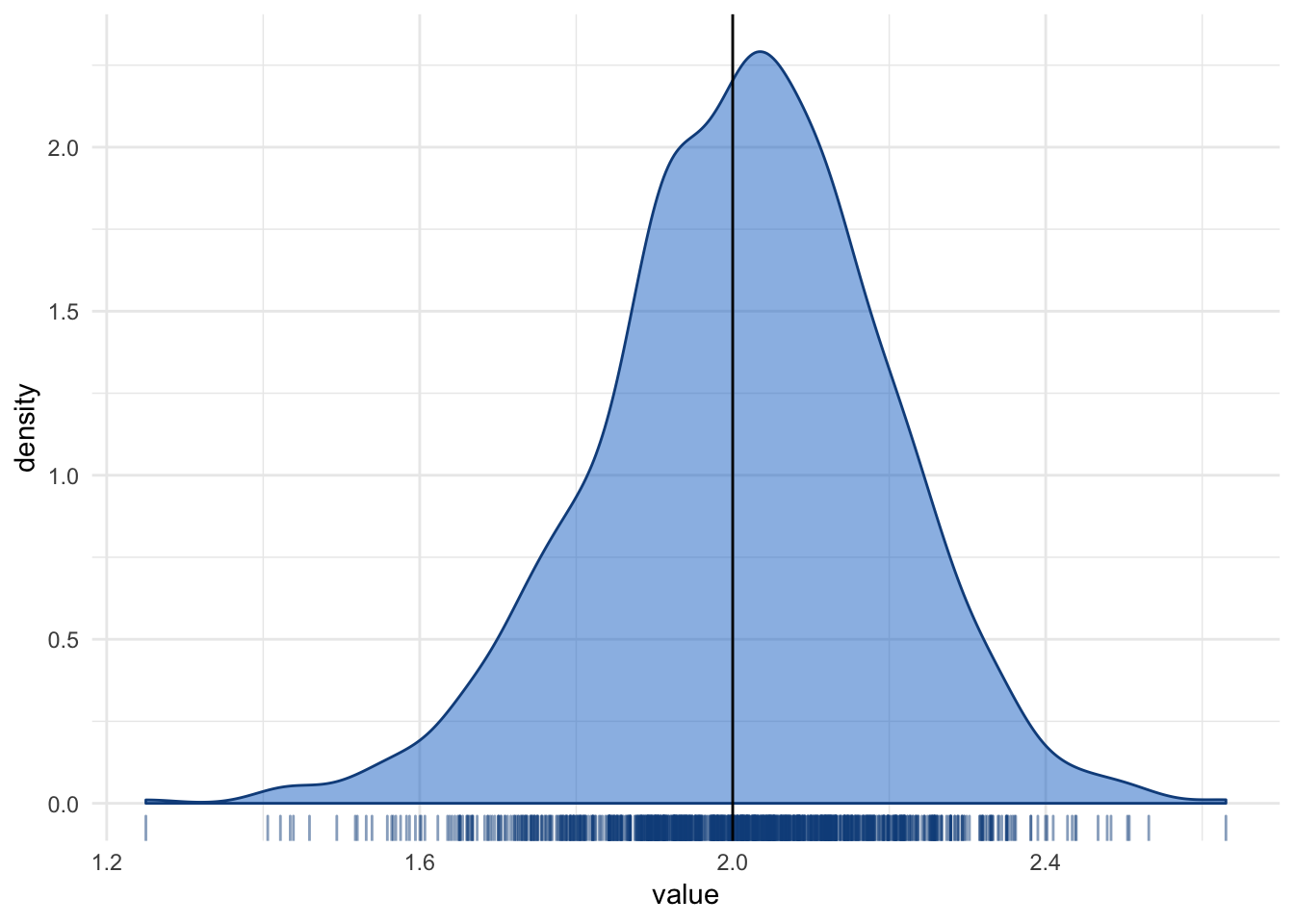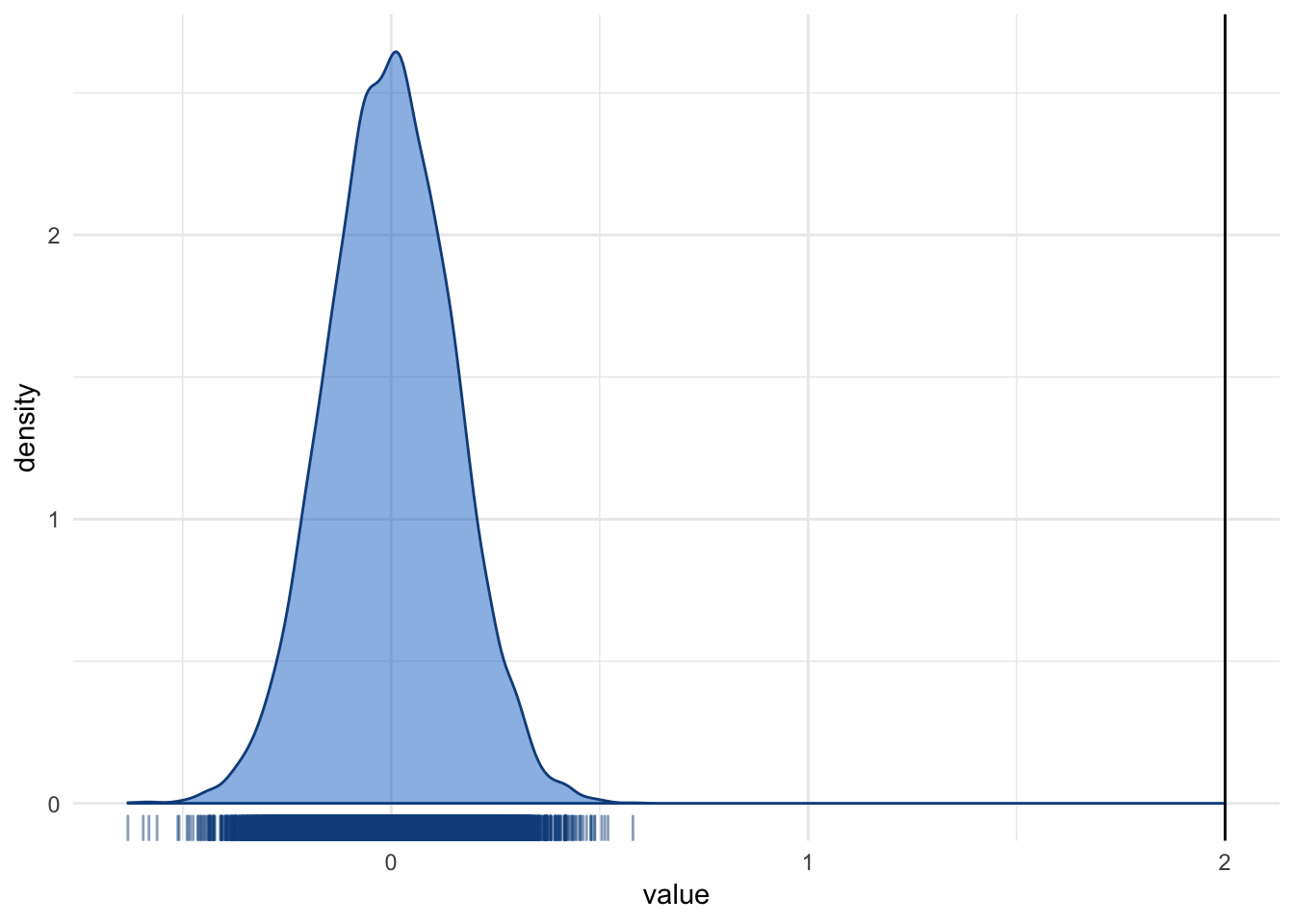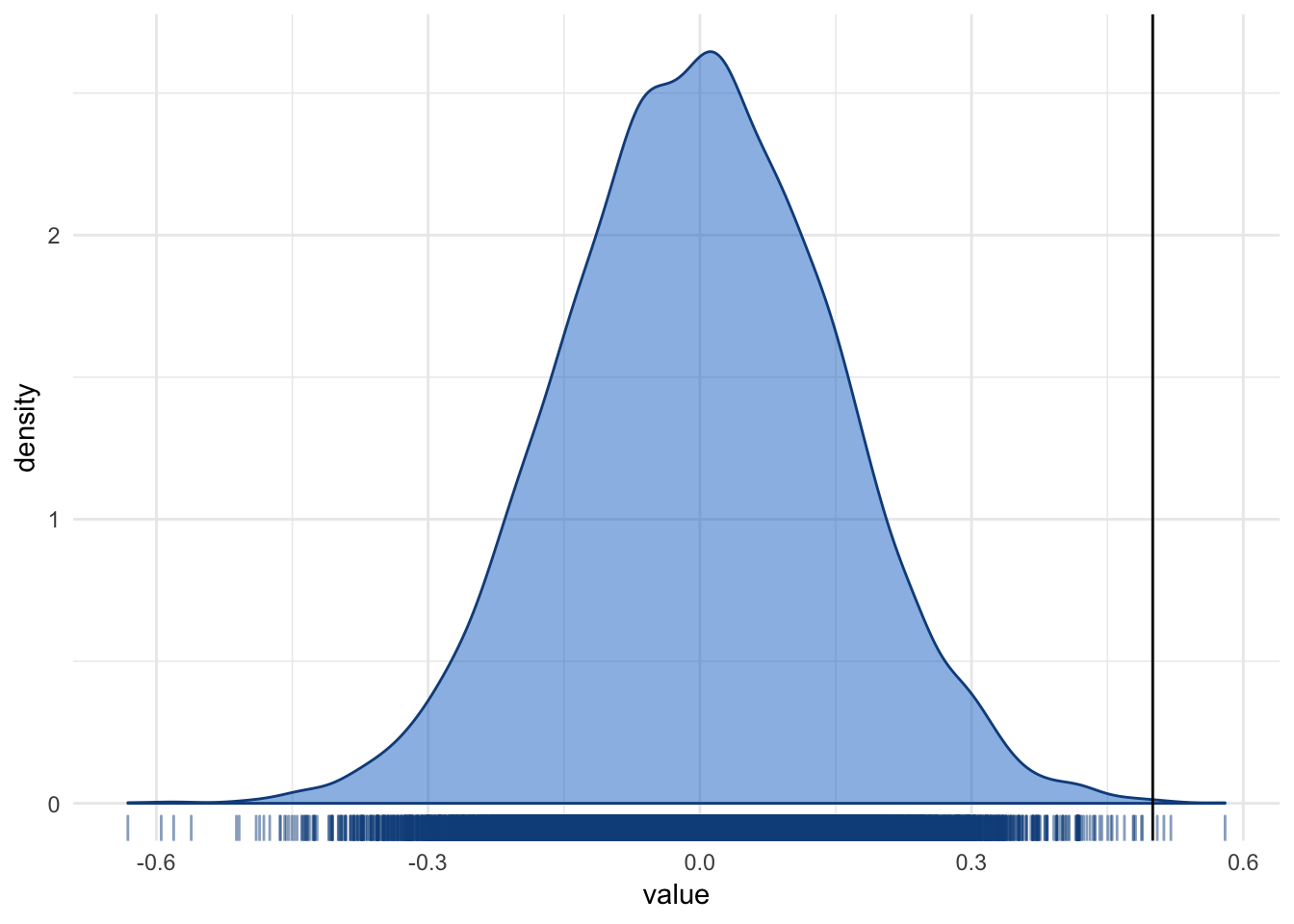set.seed(84322)
library(tidyverse)
library(boot)

# Resampling

In many cases, when assumptions of homoskedasticity do not hold, the distribution of the estimate is unknown, or when assumptions of normality may be problematic, resampling techniques can be used to draw inference instead of relying on normal theory to give us proper standard errors. Herein, we will see two common types:

1. Boostrapping
2. Monte Carlo

This is a brief introduction to both methods. A more extensive review should be conducted before its use in a scientific article.

## Boostrapping

Bootstrapping takes your data and resamples—with replacement—many times, calculating the estimate on each resample. It is best understood with an example. Let’s say we wanted to calculate the effect of age and sex on income in the population based on a sample collected. Our best guess for the effects are those observed in the sample. But we may not think that the regular standard errors would work well (possibly because of heteroskedasticity and normality issues). In this case, we can use boostrapping to get standard errors or a confidence interval instead of relying on the regular SEs and CIs. To do this, we will use boot::boot() (see documentation).

df <- data_frame(
age = rnorm(100, mean = 25, sd = 20),
sex = rbinom(100, 1, .5),
income = 2 * age * sex + runif(100, min = 0, max = 50))

est <- function(data, indices){
lm(income ~ age * sex,
data = data[indices, ]) %>%
coef(.) %>%
.     ## grabs the interaction coefficient
}

booted <- boot(data = df,
statistic = est,
R = 1000)
booted
##
## ORDINARY NONPARAMETRIC BOOTSTRAP
##
##
## Call:
## boot(data = df, statistic = est, R = 1000)
##
##
## Bootstrap Statistics :
##     original     bias    std. error
## t1* 1.999988 0.01102065   0.1821918

The output shows the estimate (original) and its standard error (std. error). Let’s see how close this is to regular regression in this case.

lm(income ~ age * sex, data = df) %>%
summary()
##
## Call:
## lm(formula = income ~ age * sex, data = df)
##
## Residuals:
##     Min      1Q  Median      3Q     Max
## -26.033 -12.012   1.803  12.027  23.411
##
## Coefficients:
##             Estimate Std. Error t value Pr(>|t|)
## (Intercept) 26.48360    3.87869   6.828  7.8e-10 ***
## age          0.03078    0.13007   0.237    0.813
## sex         -1.68730    4.71567  -0.358    0.721
## age:sex      1.99999    0.16386  12.206  < 2e-16 ***
## ---
## Signif. codes:  0 '***' 0.001 '**' 0.01 '*' 0.05 '.' 0.1 ' ' 1
##
## Residual standard error: 13.95 on 96 degrees of freedom
## Multiple R-squared:  0.8594, Adjusted R-squared:  0.855
## F-statistic: 195.6 on 3 and 96 DF,  p-value: < 2.2e-16

The standard errors in this case are very similar (likely because the assumptions of the model actually do hold here pretty well).

We can look at what the distribution of the bootstrapped samples.

booted$t %>% data.frame("value" = .) %>% ggplot(aes(value)) + geom_density(fill = "dodgerblue3", color = "dodgerblue4", alpha = .5) + geom_rug(color = "dodgerblue4", alpha = .5) + geom_vline(xintercept = booted$t0) +
theme_minimal()Each value shown in the density plot is a single interaction effect observed in a single bootstrap sample. In this case, there are 1000 individual values. From this information, the bootstrapped method calculated the standard error, assuming this distribution is approximately normal. If it is far from normal, other approaches where you calculate the confidence interval instead of the standard error are also possible.

Ultimately, bootstrapping gives you an empirical distribution of the estimate, with no major assumptions about the estimate itself.

## Monte Carlo

Monte Carlo, on the other hand, tackles the problem differently. Whereas bootstrapping makes an empirical distribution of the estimate itself, Monte Carlo establishes what the world would look like under the null hypothesis and then compares our result to that world. Using that information, we can get a p-value. Again, an example is helpful. Here, we use the same example as before but assume the null is true; that is, that the interaction effect is 0. So we calculate a bunch of data sets where the null is true.

null <- replicate(10000, {

data_frame(
age = rnorm(100, mean = 25, sd = 20),
sex = rbinom(100, 1, .5),
income = 0 * age * sex + runif(100, min = 0, max = 50)) %>%
lm(income ~ age * sex, data = .) %>%
coef(.) %>%
.

})

null %>%
data.frame("value" = .) %>%
ggplot(aes(value)) +
geom_density(fill = "dodgerblue3",
color = "dodgerblue4",
alpha = .5) +
geom_rug(color = "dodgerblue4",
alpha = .5) +
geom_vline(xintercept = booted\$t0) +
theme_minimal()We ran the Monte Carlo simulations 10,000 times and zero were past the value. That would suggest our p is far below the usual p < .001. If we run a different example where the result isn’t so extreme. In this case, our observed coefficient was, let’s say, 0.5.

null %>%
data.frame("value" = .) %>%
ggplot(aes(value)) +
geom_density(fill = "dodgerblue3",
color = "dodgerblue4",
alpha = .5) +
geom_rug(color = "dodgerblue4",
alpha = .5) +
geom_vline(xintercept = .5) +
theme_minimal()We can see that some of the values are more extreme than the observed coefficient. We can see how many of the 10,000 are more extreme than it.

sum(abs(null) > .5)
##  10

Of the 10,000 values, only 10 are more extreme than the 0.5 coefficient. That means, our p-value here is 10/10000 = 0.001.

The nice thing about Monte Carlo simulations is that you can use all sorts of distributions and conditions that are otherwise not possible.